Home > MC2 > Chapter 11 > Lesson 11.1.2 > Problem11-22

11-22.
1. Answer the comparison questions below. Homework Help ✎

1. How many times larger is 3 · 3 · 3 than 3 + 3 + 3?

2. How many times larger is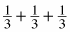than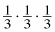?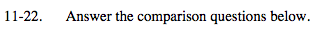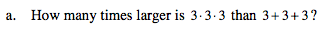What is the product of 3(3)(3)?
What is the sum of 3+3+3?

3(3)(3)=27
3+3+3=9

How do these values compare?

The product (27) is 3 times larger than the sum (9).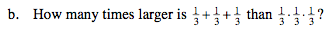Use the method in (a).